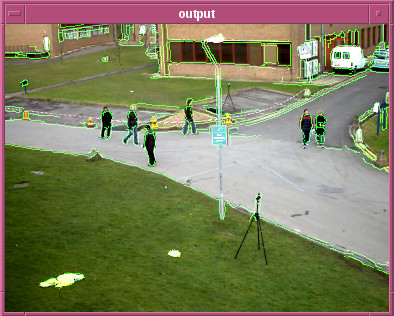OpenCV  4.5.4 Open Source Computer Vision
Graph API

# Introduction

OpenCV Graph API (or G-API) is a new OpenCV module targeted to make regular image processing fast and portable. These two goals are achieved by introducing a new graph-based model of execution.

G-API is a special module in OpenCV – in contrast with the majority of other main modules, this one acts as a framework rather than some specific CV algorithm. G-API provides means to define CV operations, construct graphs (in form of expressions) using it, and finally implement and run the operations for a particular backend.

Note
G-API is a new module and now is in active development. It's API is volatile at the moment and there may be minor but compatibility-breaking changes in the future.

# Contents

G-API documentation is organized into the following chapters:

# API Example

A very basic example of G-API pipeline is shown below:

#include <opencv2/gapi.hpp>
int main(int argc, char *argv[])
{
if (argc > 1) cap.open(argv);
else cap.open(0);
cv::GMat vga = cv::gapi::resize(in, cv::Size(), 0.5, 0.5);
cv::GMat blurred = cv::gapi::blur(gray, cv::Size(5,5));
cv::GMat edges = cv::gapi::Canny(blurred, 32, 128, 3);
cv::GMat b,g,r;
std::tie(b,g,r) = cv::gapi::split3(vga);
cv::GMat out = cv::gapi::merge3(b, g | edges, r);
cv::GComputation ac(in, out);
cv::Mat input_frame;
cv::Mat output_frame;
do
{
ac.apply(input_frame, output_frame);
cv::imshow("output", output_frame);
} while (cap.read(input_frame) && cv::waitKey(30) < 0);
return 0;
}

G-API is a separate OpenCV module so its header files have to be included explicitly. The first four lines of main() create and initialize OpenCV's standard video capture object, which fetches video frames from either an attached camera or a specified file.

G-API pipeline is constructed next. In fact, it is a series of G-API operation calls on cv::GMat data. The important aspect of G-API is that this code block is just a declaration of actions, but not the actions themselves. No processing happens at this point, G-API only tracks which operations form pipeline and how it is connected. G-API Data objects (here it is cv::GMat) are used to connect operations each other. in is an empty cv::GMat signalling that it is a beginning of computation.

After G-API code is written, it is captured into a call graph with instantiation of cv::GComputation object. This object takes input/output data references (in this example, in and out cv::GMat objects, respectively) as parameters and reconstructs the call graph based on all the data flow between in and out.

cv::GComputation is a thin object in sense that it just captures which operations form up a computation. However, it can be used to execute computations – in the following processing loop, every captured frame (a cv::Mat input_frame) is passed to cv::GComputation::apply().Example pipeline running on sample video 'vtest.avi'

cv::GComputation::apply() is a polimorphic method which accepts a variadic number of arguments. Since this computation is defined on one input, one output, a special overload of cv::GComputation::apply() is used to pass input data and get output data.

Internally, cv::GComputation::apply() compiles the captured graph for the given input parameters and executes the compiled graph on data immediately.

There is a number important concepts can be outlines with this example:

• Graph declaration and graph execution are distinct steps;
• Graph is built implicitly from a sequence of G-API expressions;
• G-API supports function-like calls – e.g. cv::gapi::resize(), and operators, e.g operator|() which is used to compute bitwise OR;
• G-API syntax aims to look pure: every operation call within a graph yields a new result, thus forming a directed acyclic graph (DAG);
• Graph declaration is not bound to any data – real data objects (cv::Mat) come into picture after the graph is already declared.

See tutorials and porting examples to learn more on various G-API features and concepts.